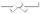# Exercises

0 / 10

Consider the following class definitions:

``public class LinkedChain<T> { private Node<T> firstNode; private int...``0 / 10

Consider the following class definitions:

``public class LinkedChain<T> { private Node<T> firstNode; private int...``0 / 10

Consider the following class definitions:

``public class LinkedChain<T> { private Node<T> firstNode; private int...``0 / 10

Consider the following class definitions:

``public class LinkedChain<T> { private Node<T> firstNode; private int...``0 / 10

question text goes here0 / 10

Consider the following class definitions:

``public class LinkedChain<T> { private Node<T> firstNode; private int...``0 / 10

Consider the following class definitions:

``public class LinkedChain<T> { private Node<T> firstNode; private int...``0 / 10

Consider the following class definitions:

``public class LinkedChain<T> { private Node<T> firstNode; private int...``0 / 10

Consider the following class definitions:

``public class LinkedChain<T> { private Node<T> firstNode; private int...``0 / 10

Consider the following class definitions:

``public class LinkedChain<T> { private Node<T> firstNode; private int...``0 / 10

Consider the following class definitions:

``public class LinkedChain<T> { private Node<T> firstNode; private int...``0 / 50

Below is some code your co-worker implemented, but they're asking you for help because it's not working the way they want it to.

The code...0 / 50

You notice some co-worker's code is both not working and is overly complicated.

The code should act in the following way:

• if value is ...0 / 50

Below is some code your co-worker implemented, but they're asking you for help because it's not working the way they want it to.

The code...0 / 50

Below is some code your co-worker tried again to implement letter grade code, but it is still not working the way they want.

The code ...0 / 50

For the question below, assume the following implementation of a Person class:

``public class Person { private int age; private String...``0 / 50

For the question below, assume the following implementation of a Person class:

``public class Person { private int age; private String...``0 / 50

For the question below, assume the following implementation of a Person class:

``public class Person { private int age; private String...``0 / 10

Consider the following class definition:
Object data;
}
Use a single assignment statement to make the variable p...0 / 10

Consider the following class definition:
Object data;
}
Use a single assigment to set the info of the Node...0 / 50

For the question below, assume the following implementation of the Ball class

``public class Ball{ private String color; private double...``0 / 50

For this question, assume the following implementation of the class Person and the following UML diagram

#### Person Class Implementation

``...``0 / 50

For this question, assume the following implementation of the class Person and the following UML diagram

#### Person Class Implementation

``...``0 / 50

Blank diagram.png0 / 50

For the question below, assume the following implementation of an Employee class:

``class Employee { String name; public Employee(String ...``